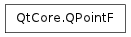QLine

QPoint

# QPointF¶## Detailed Description¶

The PySide.QtCore.QPointF class defines a point in the plane using floating point precision.

A point is specified by a x coordinate and an y coordinate which can be accessed using the PySide.QtCore.QPointF.x() and PySide.QtCore.QPointF.y() functions. The coordinates of the point are specified using floating point numbers for accuracy. The PySide.QtCore.QPointF.isNull() function returns true if both x and y are set to 0.0. The coordinates can be set (or altered) using the PySide.QtCore.QPointF.setX() and PySide.QtCore.QPointF.setY() functions, or alternatively the PySide.QtCore.QPointF.rx() and PySide.QtCore.QPointF.ry() functions which return references to the coordinates (allowing direct manipulation).

Given a point p , the following statements are all equivalent:

```p = QPointF()

p.setX(p.x() + 1.0)
p += QPointF(1.0, 0.0)
#p.rx()++;
```

A PySide.QtCore.QPointF object can also be used as a vector: Addition and subtraction are defined as for vectors (each component is added separately). A PySide.QtCore.QPointF object can also be divided or multiplied by an int or a qreal .

In addition, the PySide.QtCore.QPointF class provides a constructor converting a PySide.QtCore.QPoint object into a PySide.QtCore.QPointF object, and a corresponding PySide.QtCore.QPointF.toPoint() function which returns a PySide.QtCore.QPoint copy of this point. Finally, PySide.QtCore.QPointF objects can be streamed as well as compared.

class PySide.QtCore.QPointF
class PySide.QtCore.QPointF(p)
class PySide.QtCore.QPointF(QPointF)
class PySide.QtCore.QPointF(xpos, ypos)
Parameters: p – PySide.QtCore.QPoint ypos – PySide.QtCore.qreal xpos – PySide.QtCore.qreal QPointF – PySide.QtCore.QPointF

Constructs a null point, i.e. with coordinates (0.0, 0.0)

Constructs a copy of the given point .

Constructs a point with the given coordinates (x , y ).

PySide.QtCore.QPointF.__reduce__()
Return type: PyObject
PySide.QtCore.QPointF.__repr__()
Return type: PyObject
PySide.QtCore.QPointF.isNull()
Return type: PySide.QtCore.bool

Returns true if both the x and y coordinates are set to +0.0; otherwise returns false.

Note

Since this function treats +0.0 and -0.0 differently, points with zero-valued coordinates where either or both values have a negative sign are not defined to be null points.

PySide.QtCore.QPointF.manhattanLength()
Return type: PySide.QtCore.qreal

Returns the sum of the absolute values of PySide.QtCore.QPointF.x() and PySide.QtCore.QPointF.y() , traditionally known as the “Manhattan length” of the vector from the origin to the point.

PySide.QtCore.QPointF.__ne__(p2)
Parameters: p2 – PySide.QtCore.QPointF PySide.QtCore.bool
PySide.QtCore.QPointF.__mul__(c)
Parameters: c – PySide.QtCore.qreal PySide.QtCore.QPointF
PySide.QtCore.QPointF.__mul__(c)
Parameters: c – PySide.QtCore.qreal PySide.QtCore.QPointF
PySide.QtCore.QPointF.__mul__(m)
Parameters: m – PySide.QtGui.QTransform PySide.QtCore.QPointF
PySide.QtCore.QPointF.__mul__(matrix)
Parameters: matrix – PySide.QtGui.QMatrix4x4 PySide.QtCore.QPointF
PySide.QtCore.QPointF.__mul__(m)
Parameters: m – PySide.QtGui.QMatrix PySide.QtCore.QPointF
PySide.QtCore.QPointF.__mul__(matrix)
Parameters: matrix – PySide.QtGui.QMatrix4x4 PySide.QtCore.QPointF
PySide.QtCore.QPointF.__imul__(c)
Parameters: c – PySide.QtCore.qreal PySide.QtCore.QPointF

Multiplies this point’s coordinates by the given factor , and returns a reference to this point. For example:

```p = QPointF(-1.1, 4.1)
p *= 2.5  # p becomes (-2.75, 10.25)
```

PySide.QtCore.QPointF.operator/=()

Parameters: p2 – PySide.QtCore.QPointF PySide.QtCore.QPointF
Parameters: p – PySide.QtCore.QPointF PySide.QtCore.QPointF

Adds the given point to this point and returns a reference to this point. For example:

```p = QPointF( 3.1, 7.1)
q = QPointF(-1.0, 4.1)
p += q    # p becomes (2.1, 11.2)
```

PySide.QtCore.QPointF.operator-=()

PySide.QtCore.QPointF.__sub__()
Return type: PySide.QtCore.QPointF
PySide.QtCore.QPointF.__sub__(p2)
Parameters: p2 – PySide.QtCore.QPointF PySide.QtCore.QPointF
PySide.QtCore.QPointF.__isub__(p)
Parameters: p – PySide.QtCore.QPointF PySide.QtCore.QPointF

Subtracts the given point from this point and returns a reference to this point. For example:

```p = QPointF( 3.1, 7.1)
q = QPointF(-1.0, 4.1)
p -= q    # p becomes (4.1, 3.0)
```

PySide.QtCore.QPointF.operator+=()

PySide.QtCore.QPointF.__div__(c)
Parameters: c – PySide.QtCore.qreal PySide.QtCore.QPointF
PySide.QtCore.QPointF.__idiv__(c)
Parameters: c – PySide.QtCore.qreal PySide.QtCore.QPointF

Divides both x and y by the given divisor , and returns a reference to this point. For example:

```p = QPointF(-2.75, 10.25)
p /= 2.5  # p becomes (-1.1, 4.1)
```

PySide.QtCore.QPointF.operator*=()

PySide.QtCore.QPointF.__eq__(p2)
Parameters: p2 – PySide.QtCore.QPointF PySide.QtCore.bool
PySide.QtCore.QPointF.setX(x)
Parameters: x – PySide.QtCore.qreal

Sets the x coordinate of this point to the given x coordinate.

PySide.QtCore.QPointF.setY(y)
Parameters: y – PySide.QtCore.qreal

Sets the y coordinate of this point to the given y coordinate.

PySide.QtCore.QPointF.toPoint()
Return type: PySide.QtCore.QPoint

Rounds the coordinates of this point to the nearest integer, and returns a PySide.QtCore.QPoint object with the rounded coordinates.

PySide.QtCore.QPointF.QPointF()

PySide.QtCore.QPointF.toTuple()
Return type: PyObject
PySide.QtCore.QPointF.x()
Return type: PySide.QtCore.qreal

Returns the x-coordinate of this point.